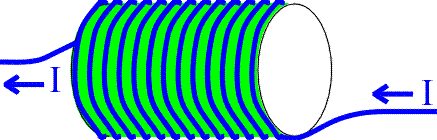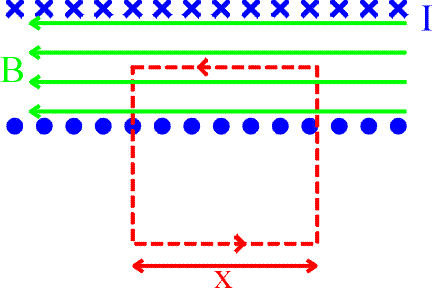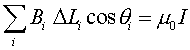Magnetic field of a solenoidA solenoid is a coil of wire designed to create a strong magnetic field inside the coil. By wrapping the same wire many times around a cylinder, the magnetic field due to the wires can become quite strong. The number of turns N refers to the number of loops the solenoid has. More loops will bring about a stronger magnetic field. The formula for the field inside the solenoid is

B = m0 I N / L

This formula can be accepted on faith; or it can be derived using Ampere's law as follows. Look at a cross section of the solenoid.The blue crosses represent the current traveling into the page, while the blue dots represent the currents coming out of the page. Ampere's law (left) for the red path can be written as.where the number of loops enclose by the path is (N/L)x. Only the upper portion of the path contributed to the sum because the magnetic field is zero outside, and because the vertical paths are perpendicular to the magnetic field. By dividing x out of both sides of the last equation, one finds:The magnetic field inside a solenoid is proportional to both the applied current and the number of turns per unit length. There is no dependence on the diameter of the solenoid, and the field strength doesn't depend on the position inside the solenoid, i.e., the field inside is constant.

Examples       Magnetic field sources index        Lecture index